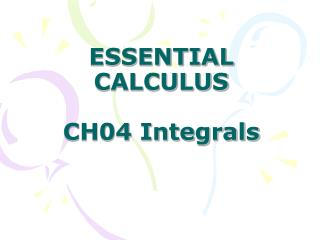DownloadDownload PresentationESSENTIAL CALCULUS CH04 Integrals

# ESSENTIAL CALCULUS CH04 Integrals

Télécharger la présentation## ESSENTIAL CALCULUS CH04 Integrals

- - - - - - - - - - - - - - - - - - - - - - - - - - - E N D - - - - - - - - - - - - - - - - - - - - - - - - - - -
##### Presentation Transcript

1. ESSENTIAL CALCULUSCH04 Integrals

2. In this Chapter: • 4.1 Areas and Distances • 4.2 The Definite Integral • 4.3 Evaluating Definite Integrals • 4.4 The Fundamental Theorem of Calculus • 4.5 The Substitution Rule Review

3. Chapter 4, 4.1, P194

4. Chapter 4, 4.1, P195

5. Chapter 4, 4.1, P195

6. Chapter 4, 4.1, P195

7. Chapter 4, 4.1, P195

8. Chapter 4, 4.1, P195

9. Chapter 4, 4.1, P196

10. Chapter 4, 4.1, P197

11. Chapter 4, 4.1, P197

12. Chapter 4, 4.1, P198

13. Chapter 4, 4.1, P198

14. Chapter 4, 4.1, P199

15. 2. DEFINITION The area A of the region S that lies under the graph of the continuous function f is the limit of the sum of the areas of approximating rectangles: A=lim Rn=lim[f(x1)∆x+f(x2) ∆x+‧‧‧+f(xn) ∆x] n→∞ n→∞ Chapter 4, 4.1, P199

16. Chapter 4, 4.1, P199

17. This tells us to end with i=n. This tells us to add. This tells us to start with i=m. Chapter 4, 4.1, P199

18. Chapter 4, 4.1, P199

19. The area of A of the region S under the graphs of the continuous function f is A=lim[f(x1)∆x+f(x2) ∆x+‧‧‧+f(xn) ∆x] A=lim[f(x0)∆x+f(x1) ∆x+‧‧‧+f(xn-1) ∆x] A=lim[f(x*1)∆x+f(x*2) ∆x+‧‧‧+f(x*n) ∆x] n→∞ n→∞ n→∞ Chapter 4, 4.1, P200

20. FIGURE 1 A partition of [a,b] with sample points Chapter 4, 4.2, P205

21. A Riemann sum associated with a partition P and a function f is constructed by evaluating f at the sample points, multiplying by the lengths of the corresponding subintervals, and adding: Chapter 4, 4.2, P205

22. FIGURE 2 A Riemann sum is the sum of the areas of the rectangles above the x-axis and the negatives of the areas of the rectangles below the x-axis. Chapter 4, 4.2, P206

23. 2. DEFINITION OF A DEFINITE INTEGRAL If f is a function defined on [a,b] ,the definite integral of f from a to b is the number provided that this limit exists. If it does exist, we say that f is integrable on [a,b] . Chapter 4, 4.2, P206

24. NOTE 1 The symbol ∫was introduced by Leibniz and is called an integral sign. It is an elongated S and was chosen because an integral is a limit of sums. In the notation is called the integrand and a and b are called the limits of integration; a is the lower limit and b is the upper limit. The symbol dx has no official meaning by itself; is all one symbol. The procedure of calculating an integral is called integration. Chapter 4, 4.2, P206

25. Chapter 4, 4.2, P206

26. 3. THEOREM If f is continuous on [a,b], or if f has only a finite number of jump discontinuities, then f is integrable on [a,b]; that is, the definite integral dx exists. Chapter 4, 4.2, P207

27. 4. THEOREM If f is integrable on [a,b], then where Chapter 4, 4.2, P207

28. Chapter 4, 4.2, P208

29. Chapter 4, 4.2, P208

30. Chapter 4, 4.2, P208

31. Chapter 4, 4.2, P208

32. Chapter 4, 4.2, P210

33. Chapter 4, 4.2, P211

34. MIDPOINT RULE where and Chapter 4, 4.2, P211

35. Chapter 4, 4.2, P212

36. Chapter 4, 4.2, P212

37. Chapter 4, 4.2, P213

38. Chapter 4, 4.2, P213

39. PROPERTIES OF THE INTEGRAL Suppose all the following integrals exist. where c is any constant where c is any constant Chapter 4, 4.2, P213

40. Chapter 4, 4.2, P214

41. Chapter 4, 4.2, P214

42. COMPARISON PROPERTIES OF THE INTEGRAL 6. If f(x)≥0 fpr a≤x≤b. then 7.If f(x)≥g(x) for a≤x≤b, then 8.If m≤f(x) ≤M for a≤x≤b, then Chapter 4, 4.2, P214

43. Chapter 4, 4.2, P215

44. 29.The graph of f is shown. Evaluate each integral by interpreting it in terms of areas. • (b) • (c) (d) Chapter 4, 4.3, P217

45. 30. The graph of g consists of two straight lines and a semicircle. Use it to evaluate each integral. (a) (b) (c) Chapter 4, 4.3, P217

46. EVALUATION THEOREM If f is continuous on the interval [a,b] , then Where F is any antiderivative of f, that is, F’=f. Chapter 4, 4.3, P218

47. the notation ∫f(x)dx is traditionally used for an antiderivative of f and is called an indefinite integral. Thus The connection between them is given by the Evaluation Theorem: If f is continuous on [a,b], then Chapter 4, 4.3, P220

48. ▓You should distinguish carefully between definite and indefinite integrals. A definite integral is a number, whereas an indefinite integral is a function (or family of functions). Chapter 4, 4.3, P220

49. 1. TABLE OF INDEFINITE INTEGRALS Chapter 4, 4.3, P220

50. ■ Figure 3 shows the graph of the integrand in Example 5. We know from Section 4.2 that the value of the integral can be interpreted as the sum of the areas labeled with a plus sign minus the area labeled with a minus sign. Chapter 4, 4.3, P221